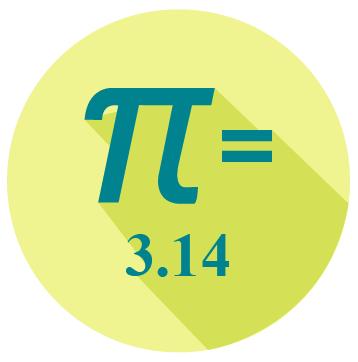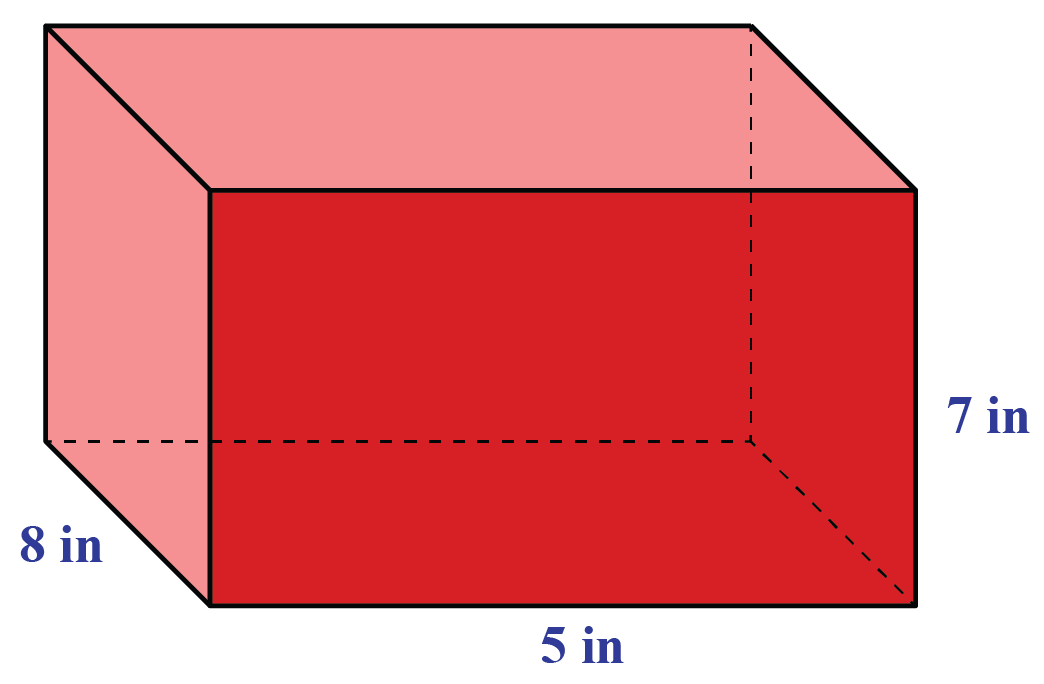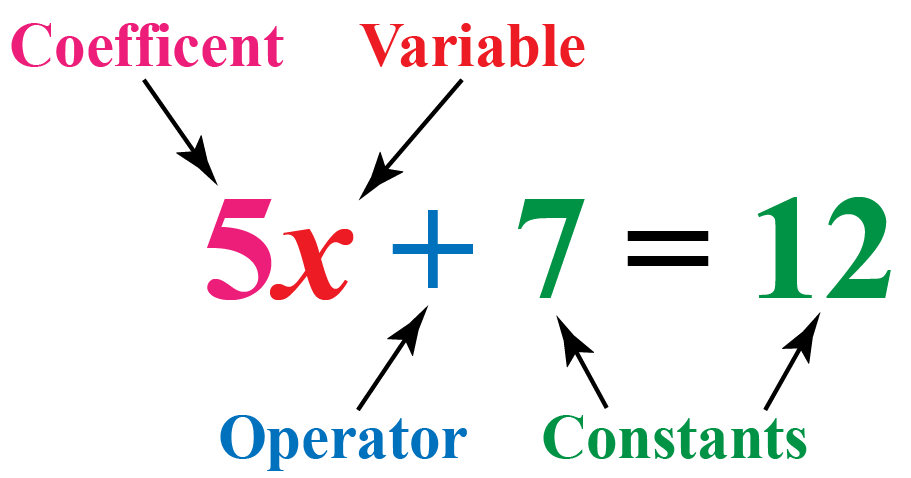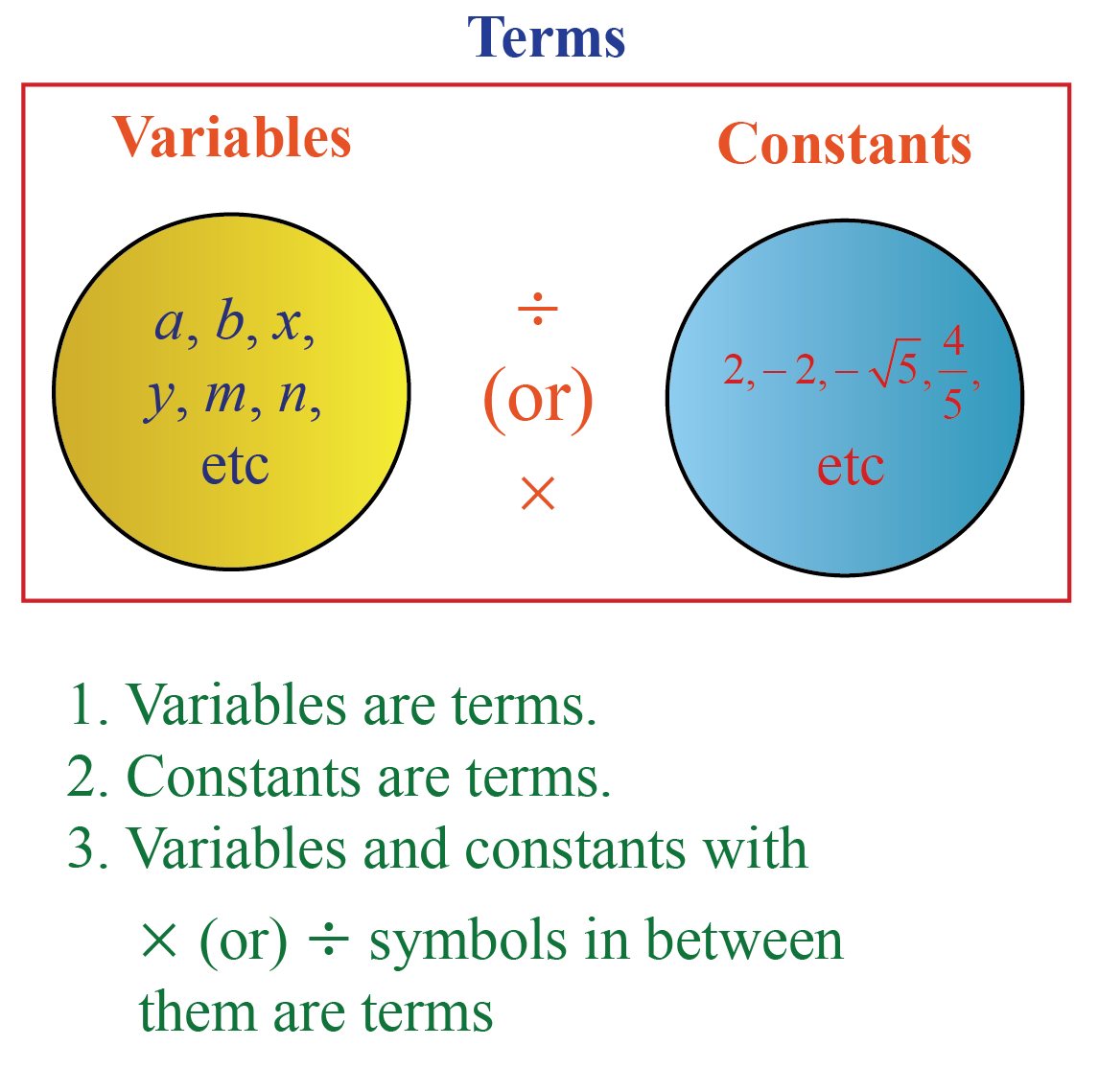# Constants

Constants

Do you know the value of $$pi$$?Can $$\pi$$ take any other value? No.

This implies that $$\pi$$ is constant because it does not change.

Can the speed of light change? No, it cannot.

The speed of light is a fixed value.

In this mini-lesson, let us explore the concept of constants in maths along with:

• constants examples
• constant and variable properties

## Lesson Plan

 1 What Is a Constant? 2 Tips and Tricks 3 Thinking Out of the Box! 4 Solved Examples on Constants 5 Interactive Questions on Constants

## What Is a Constant?

Constant is said to be the entity whose value is always fixed irrespective of the change in its usage.

These values do not change even after the change in expression.

Constants are numerical values like a natural number, whole number, real number, integer, or decimal number.

Variable is an entity whose value isn't fixed and changes as per the change in its usage.

Let us see some real-life constants examples.

• The shoe size of a 40-year-old man will not change. It remains constant.
• The number of fingers in your hand will never change. It remains constant.
• The number of your eyes will always be two.## Difference Between Constants and Variables

Constants Variables

The value of a constant does not change. It remains fixed.

The value of the variable changes according to its usage. It varies.

Constants are mostly numbers.

Variables are denoted by letters or symbols.

The value of a constant is always known.

The value of a variable is unknown.

Examples

1. The dimensions of a glass rectangular prism are:

• Length (l) = 8 inches
• Breadth (b) =  5 inches
• Height (h) = 7 inches

The dimensions of the prism are constants because they do not vary and are fixed values.2. The date on our calendar is changing every day.

Therefore, it is a variable.Tips and Tricks

• A key understanding of constants in a situation is to see the quantities which do not get changed as soon as anything in the given situation varies because these constants are the ones that do not cause any changes in the output as well.
• The key constant in any given situation is the one that does not affect the output.

## What Are Variables and Constants in an Algebraic Expression?

An algebraic expression is a mathematical expression that consists of variables, constants, coefficients, and mathematical operators.

Let us consider an example to understand better.Variables are generally denoted by letters or symbols, whereas constants are numbers.Think Tank
• Can imaginary numbers be constants?
• Is the product of a constant and a variable a constant?

## Solved Examples

 Example 1

Mathew was asked to identify the constant terms in the following expressions.

Expression 1:

$$2x +7 = 4y + 3$$

Expression 2:

$$y + 4 = x$$

Solution

Simplify the first expression.

\begin{align}2x+7&=4y+3\\4y-2x&=7-3\\4y-2x&=4 \end{align}

The constant in expression 1 is 4

Now, consider the second expression $$y+4=x$$

The constant in expression 2 is also 4

 $$\therefore$$ The constant term in both the expressions is 4
 Example 2

Billy went to a shop to buy a pair of shoes for school. After 2 years, he went to the same shop to buy a new pair of clothes. Which are the constants and variables in this statistical scenario?Solution

The shop is a constant in the above scenario. But the age of Billy, the height of Billy are variables in the above scenario.

 $$\therefore$$ Age and height of Billy are variables and shop is a constant.
 Example 3

Tim has $$5$$ apples. Jacob has 4 times more apples than Tim.

Mike has  $$x$$ more apples than Jacob.

Can you tell the exact number of apples each one of them has?

Solution

The number of apples with Tim is $$5$$.

Jacob has 4 times more apples than Tim.

Therefore, Jacob has $$4\times 5=20$$ apples.

Mike has $$x$$ more apples than Jacob.

Therefore, Mike has $$20+x$$ apples.

Since $$x$$ is unknown, the number of Mike's apples cannot be determined.

We can tell the exact number of Tim's and Jacob's apples.

 $$\therefore$$ Tim has 5 apples and Jacob has 20 apples.

## Interactive Questions

Here are a few activities for you to practice.

## Let's Summarize

We hope you enjoyed learning about Constants with the simulations and practice questions. Now, you will be able to easily solve problems on constants in math with multiple examples and scenarios you learned today.

At Cuemath, our team of math experts is dedicated to making learning fun for our favorite readers, the students!

Through an interactive and engaging learning-teaching-learning approach, the teachers explore all angles of a topic.

Be it worksheets, online classes, doubt sessions, or any other form of relation, it’s the logical thinking and smart learning approach that we, at Cuemath, believe in.

For example, in $$4x+7y=8$$, 4 and 7 are coefficients.